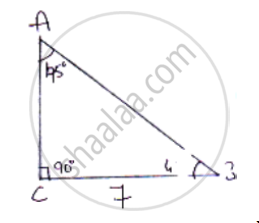Share

# In δAbc is a Right Triangle Such that ∠C = 90° ∠A = 45°, Bc = 7 Units Find ∠B, Ab and Ac - CBSE Class 10 - Mathematics

#### Question

In ΔABC is a right triangle such that ∠C = 90° ∠A = 45°, BC = 7 units find ∠B, AB and AC

#### Solution

Sum of angles in Δle = 180°

∠A + ∠B + ∠C = 180°

45° + ∠B + 90° = 180°

∠B = 180° − 135°

∠B = 45°From figure cos B = (BC)/(AB)

cos 45^2 =  7/(AB)

1/sqrt2 .  7/(AB)

AB = 7sqrt2 units

From figure sin B = (AC)/(AB)

sin 45^@ = (AB)/(7sqrt2)

1/sqrt2 = (AC)/(7sqrt2)

∴ AC =  7 units

Is there an error in this question or solution?

#### APPEARS IN

Solution In δAbc is a Right Triangle Such that ∠C = 90° ∠A = 45°, Bc = 7 Units Find ∠B, Ab and Ac Concept: Trigonometric Ratios.
S